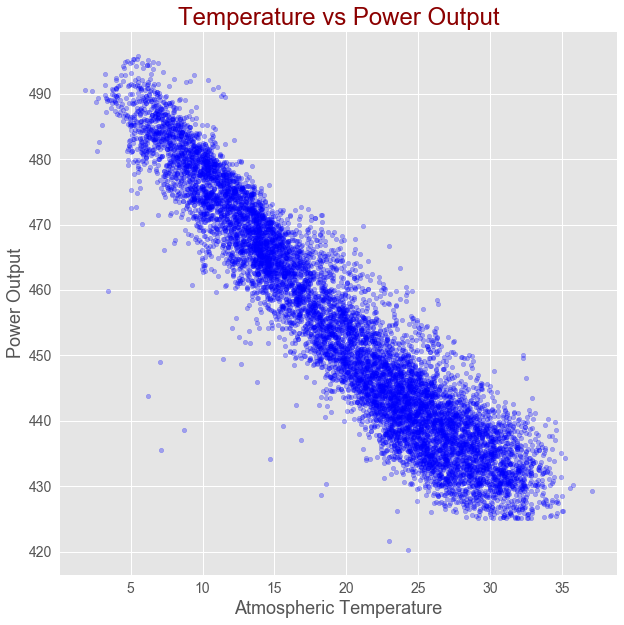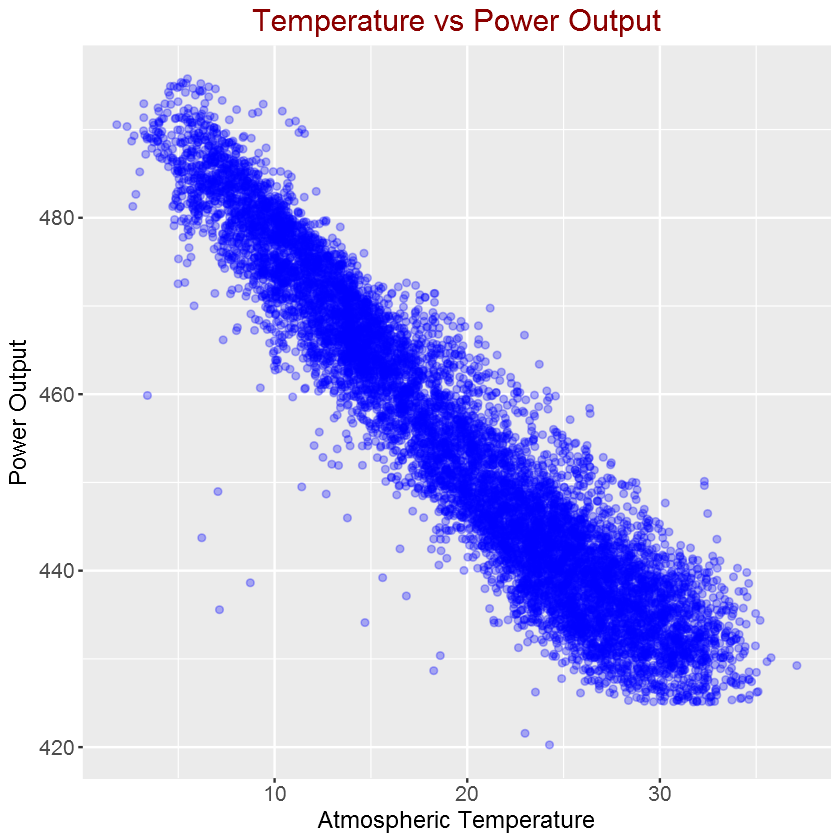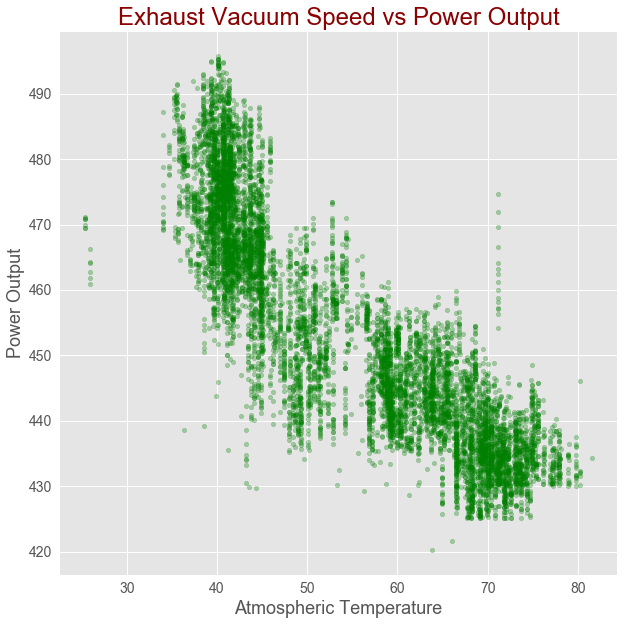# Fisseha Berhane, PhD

#### Data Scientist443-970-2353[email protected] CV Resume## Machine Learning with Python scikit-learn Vs R Caret - Part 1

This blog post series is on machine learning with Python and R. We will use the Scikit-learn library in Python and the Caret package in R. In this part, we will first perform exploratory Data Analysis (EDA) on a real-world dataset, and then apply non-regularized linear regression to solve a supervised regression problem on the dataset. We will predict power output given a set of environmental readings from various sensors in a natural gas-fired power generation plant. In the second part of the post, we will work with regularized linear regression models (ridge, lasso and elasticnet). Next, we will see the other non-linear regression models.

The real-world data we are using in this post consists of 9,568 data points, each with 4 environmental attributes collected from a Combined Cycle Power Plant over 6 years (2006-2011), and is provided by the University of California, Irvine at UCI Machine Learning Repository Combined Cycle Power Plant Data Set. You can find more details about the dataset on the UCI page. The task is a regression problem since the label (or target) we are trying to predict is numeric.

In :

### R Caret¶

Import libraries to perfrom Extract-Transform-Load (ETL) and Exploratory Data Analysis (EDA)

The caret package (short for _C_lassification _A_nd _RE_gression _T_raining) is a set of functions that attempt to streamline the process for creating predictive models.

In :
import pandas as pd
import seaborn as sns
import statsmodels.api as sm
import matplotlib.pyplot as plt
plt.style.use('ggplot') # if you are an R user and want to feel at home

In :
library(readxl)
library(ggplot2)
library(corrplot)
library(tidyverse)


In :
power_plant = pd.read_excel("Folds5x2_pp.xlsx")

In :
power_plant = read_excel("Folds5x2_pp.xlsx")


Exploratory Data Analysis (EDA)

This is a step that we should always perform before trying to fit a model to the data, as this step will often lead to important insights about our data.

In :
type(power_plant)

Out:
pandas.core.frame.DataFrame
In :
class(power_plant)

1. 'tbl_df'
2. 'tbl'
3. 'data.frame'
In :
# See first few rows


Out:
AT V AP RH PE
0 14.96 41.76 1024.07 73.17 463.26
1 25.18 62.96 1020.04 59.08 444.37
2 5.11 39.40 1012.16 92.14 488.56
3 20.86 57.32 1010.24 76.64 446.48
4 10.82 37.50 1009.23 96.62 473.90
In :
# Caret faces problems working with tbl,
# so let's change the data to simple data frame

power_plant = data.frame(power_plant)
message("The class is now ", class(power_plant))

# See first few rows

The class is now data.frame

ATVAPRHPE
14.96 41.76 1024.0773.17 463.26
25.18 62.96 1020.0459.08 444.37
5.11 39.40 1012.1692.14 488.56
20.86 57.32 1010.2476.64 446.48
10.82 37.50 1009.2396.62 473.90
26.27 59.44 1012.2358.77 443.67

The columns in the DataFrame are:

AT = Atmospheric Temperature in C

V = Exhaust Vacuum Speed

AP = Atmospheric Pressure

RH = Relative Humidity

PE = Power Output

Power Output is the value we are trying to predict given the measurements above.

In :
# Size of  DataFrame

power_plant.shape   # we have 9568 rows and 5 columns

Out:
(9568, 5)
In :
dim(power_plant)

# we have 9568 rows and 5 columns

1. 9568
2. 5
In :
# class of each column in the DataFrame

power_plant.dtypes    # all columns are numeric

Out:
AT    float64
V     float64
AP    float64
RH    float64
PE    float64
dtype: object
In :
map(power_plant, class)

# all columns are numeric

$AT 'numeric'$V
'numeric'
$AP 'numeric'$RH
'numeric'
$PE 'numeric' In : # Are there any missing values in any of the columns? power_plant.info() # There is no missing data in all of the columns  <class 'pandas.core.frame.DataFrame'> RangeIndex: 9568 entries, 0 to 9567 Data columns (total 5 columns): AT 9568 non-null float64 V 9568 non-null float64 AP 9568 non-null float64 RH 9568 non-null float64 PE 9568 non-null float64 dtypes: float64(5) memory usage: 373.8 KB  In : # Check for missing values map(power_plant, ~sum(is.na(.))) # using purrr package # There is no missing data in all of the columns $AT
0
$V 0$AP
0
$RH 0$PE
0

Visualize relationship between variables

Before we perform any modeling, it is a good idea to explore correlations between the predictors and the predictand. This step can be important as it helps us to select appropriate models. If our features and the outcome are linearly related, we may start with linear regression models. However, if the relationships between the label and the features are non-linear, non-linear ensemble models such as random forest can be better.

In :
# Correlation between power output and temperature

power_plant.plot(x ='AT', y = 'PE', kind ="scatter",
figsize = [10,10],
color ="b", alpha = 0.3,
fontsize = 14)
plt.title("Temperature vs Power Output",
fontsize = 24, color="darkred")

plt.xlabel("Atmospheric Temperature", fontsize = 18)

plt.ylabel("Power Output", fontsize = 18)

plt.show()In :
# Correlation between atmospheric temperature and
# power output

power_plant %>% ggplot(aes(AT, PE)) +
geom_point(color= "blue", alpha = 0.3) +
ggtitle("Temperature vs Power Output") +
xlab("Atmospheric Temperature") +
ylab("Power Output") +
theme(plot.title = element_text(color="darkred",
size=18,hjust = 0.5),
axis.text.y = element_text(size=12),
axis.text.x = element_text(size=12,hjust=.5),
axis.title.x = element_text(size=14),
axis.title.y = element_text(size=14))As shown in the above figure, there is strong linear correlation between Atmospheric Temperature and Power Output.

In :
# Correlation between Exhaust Vacuum Speed and power output

power_plant.plot(x ='V', y = 'PE',kind ="scatter",
figsize = [10,10],
color ="g", alpha = 0.3,
fontsize = 14)

plt.title("Exhaust Vacuum Speed vs Power Output", fontsize = 24, color="darkred")

plt.xlabel("Atmospheric Temperature", fontsize = 18)

plt.ylabel("Power Output", fontsize = 18)

plt.show()In :
# Correlation between Exhaust Vacuum Speed
# and power output

power_plant %>% ggplot(aes(V, PE)) +
geom_point(color= "darkgreen", alpha = 0.3) +
ggtitle("Exhaust Vacuum Speed vs Power Output") +
xlab("Exhaust Vacuum Speed") +
ylab("Power Output") +
theme(plot.title = element_text(color="darkred",size=18,hjust = 0.5),
axis.text.y = element_text(size=12),
axis.text.x = element_text(size=12,hjust=.5),
axis.title.x = element_text(size=14),
axis.title.y = element_text(size=14))